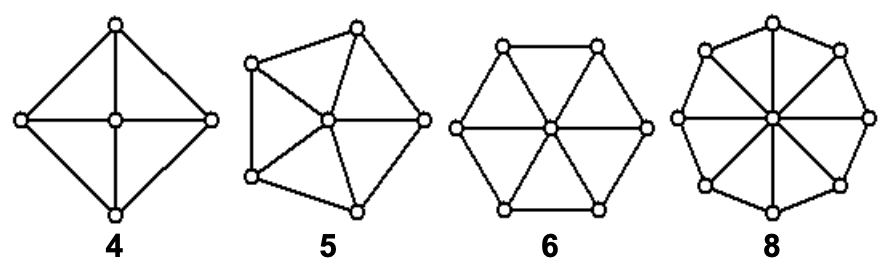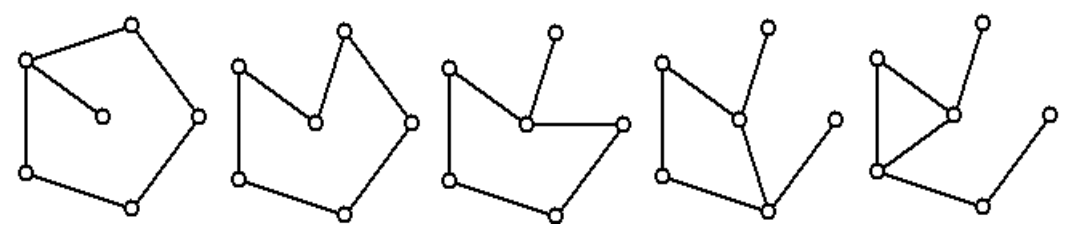시간 제한 메모리 제한 제출 정답 맞은 사람 정답 비율
1 초 512 MB 6 4 3 100.000%

## 문제

A Wheel Graph of size n is a cycle of n vertices each of which is connected to a center vertex. Examples of wheel graphs of size 4,5,6 and 8 are shown below:A spanning unicycle in a graph, G, is a spanning tree in G with one additional edge added to form a single cycle. Each of the examples below is a spanning unicycle in a wheel graph of size 5:Write a program to compute the number of different unicycles in a wheel graph of size n. Recall that two subgraphs, S1 and S2, of a graph G are different if there is at least one edge of G that is in S1 and not in S2 OR an edge in S2 which is not in S1.

## 입력

Input consists of a single line that contains a decimal integer, m (3 <= m <= 4000), which is the size of the wheel graph to find the number of unicycles of.

## 출력

The single output line consists of the count of unicycles modulo 100007 for the input size m.

## 예제 입력 1

5


## 예제 출력 1

170


## 예제 입력 2

1234


## 예제 출력 2

17511